# Chapter 9 Areas of Parallelograms and Triangles Notes for Class 9th Maths

0
1126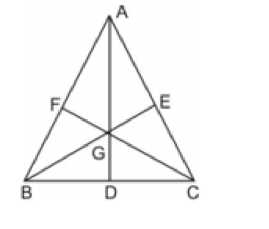Chapter 9 Areas of Parallelograms and Triangles

1. Figures on the same Base and Between the same Parallels
2. Parallelograms on the same Base and between the same Parallels
3. Triangles on the same Base and between the same Parallels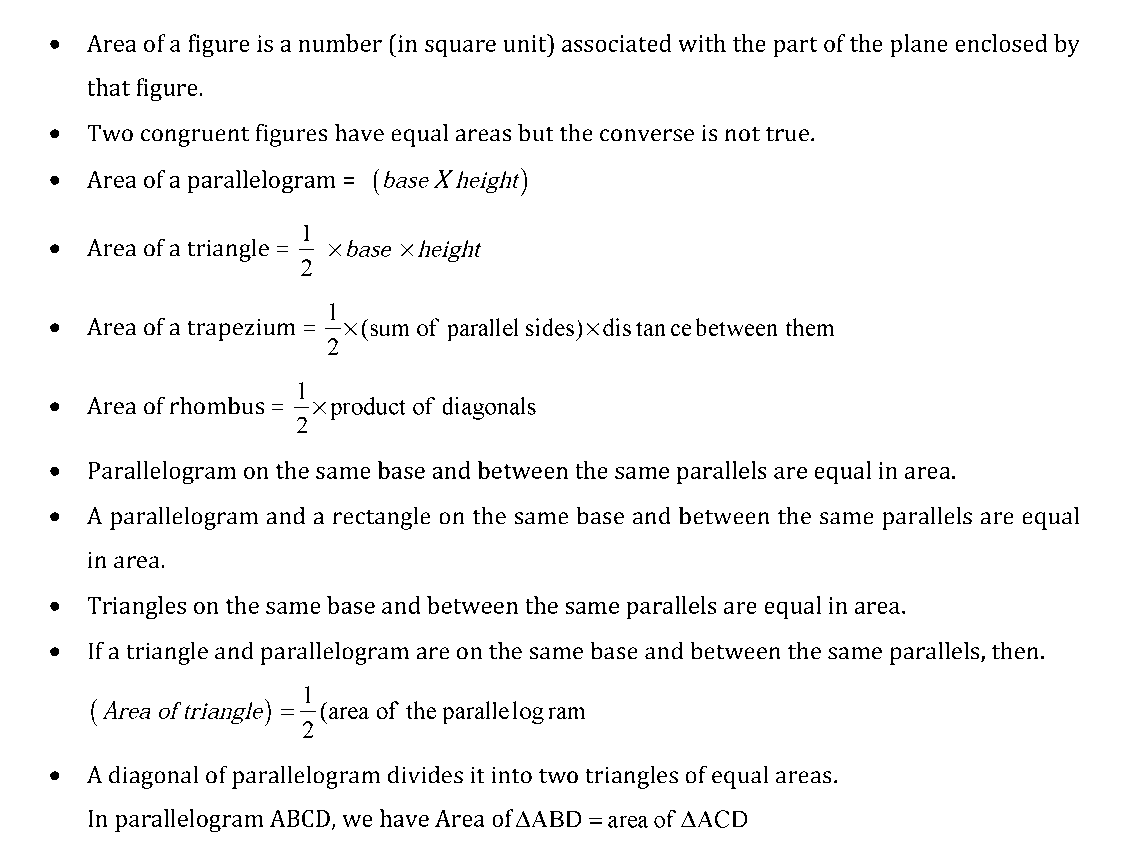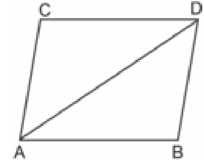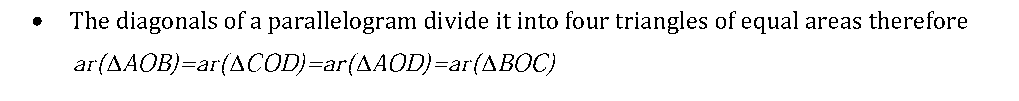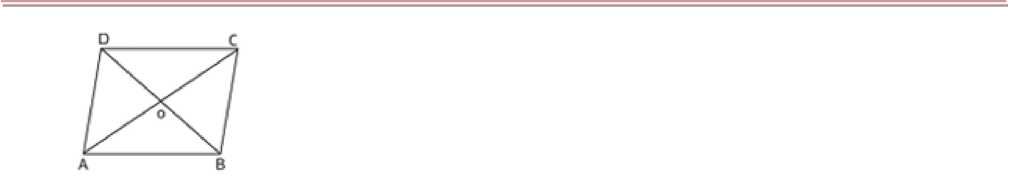• If a parallelogram and a triangle are on the same base and between the same parallel, then

area of the triangle is equal to one half area of the parallelogram.

• A median AD of a AABC divides it into two triangles of equal areas. Therefore ar(AABD)=ar(ACD )
• If the medians of a intersect at G, then ar(AAGB)=ar(AAGC )=ar(ABGC )=1 ar (AABC)• Triangles with equal bases and equal areas have equal corresponding altitude.
Previous articleChapter 8 Quadrilaterals Notes for Class 9th Maths
Next articleChapter 10 Circles Notes for Class 9th Maths